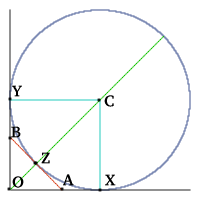#### You may also like### Polycircles

Show that for any triangle it is always possible to construct 3 touching circles with centres at the vertices. Is it possible to construct touching circles centred at the vertices of any polygon?### Circumspection

M is any point on the line AB. Squares of side length AM and MB are constructed and their circumcircles intersect at P (and M). Prove that the lines AD and BE produced pass through P.### Lawnmower

A kite shaped lawn consists of an equilateral triangle ABC of side 130 feet and an isosceles triangle BCD in which BD and CD are of length 169 feet. A gardener has a motor mower which cuts strips of grass exactly one foot wide and wishes to cut the entire lawn in parallel strips. What is the minimum number of strips the gardener must mow?

# Some(?) of the Parts

##### Age 14 to 16 Challenge Level:

Jeremy from Nottingham High School was the first to crack this one with the neatest proof that the diameter of the circle is equal to the perimeter of the triangle $OAB$ and Peter from Konstanz sent a similar proof. Other good proofs came in from Hannah and Sarah of St Philomena's School, Carshalton, Steven Cunnane of Norwich School, and James of The Robert Smyth School, Market Harborough.

If you take $OA$ as 1 unit then the perimeter $OAB$ and the diameter of the circle are equal to $2 + \sqrt{2}$ units.

Let the circle touch $OA$, $OB$ and $AB$ at $X$, $Y$ and $Z$ respectively.

Let $C$ be the centre of the circle and $R$ the radius. It is easy to prove that $OYCX$ is a square.

Then $AZ = AX$, since the tangents from a point to a circle are equal. Similarly, $BZ = BY$.The perimeter of the triangle is
$OA + OB + AB$
$= OA + OB + AZ + BZ$
$= OA + OB + AX + BY$
$= OX + OY$
$= 2R$.